# 今天你喝水了吗？喵~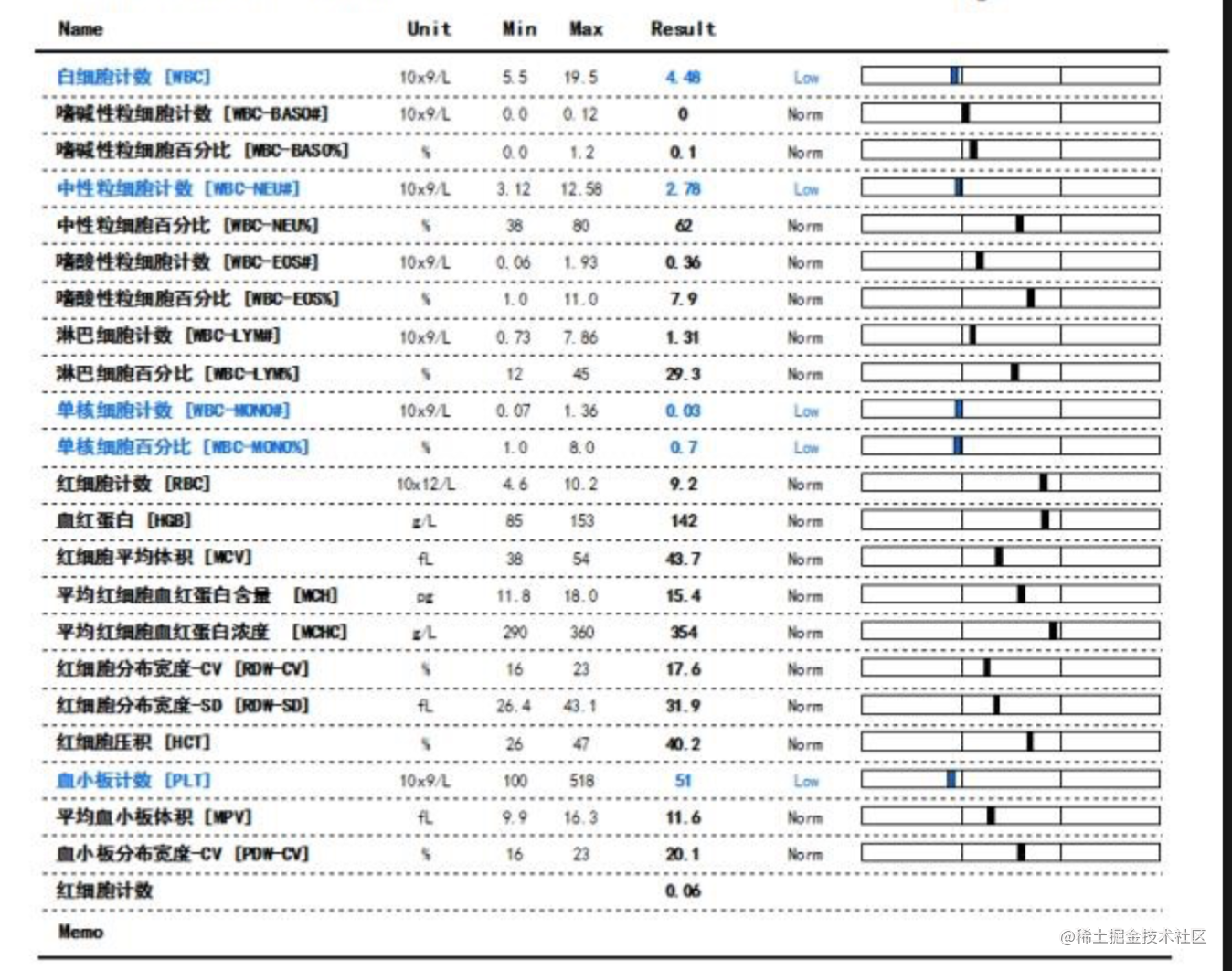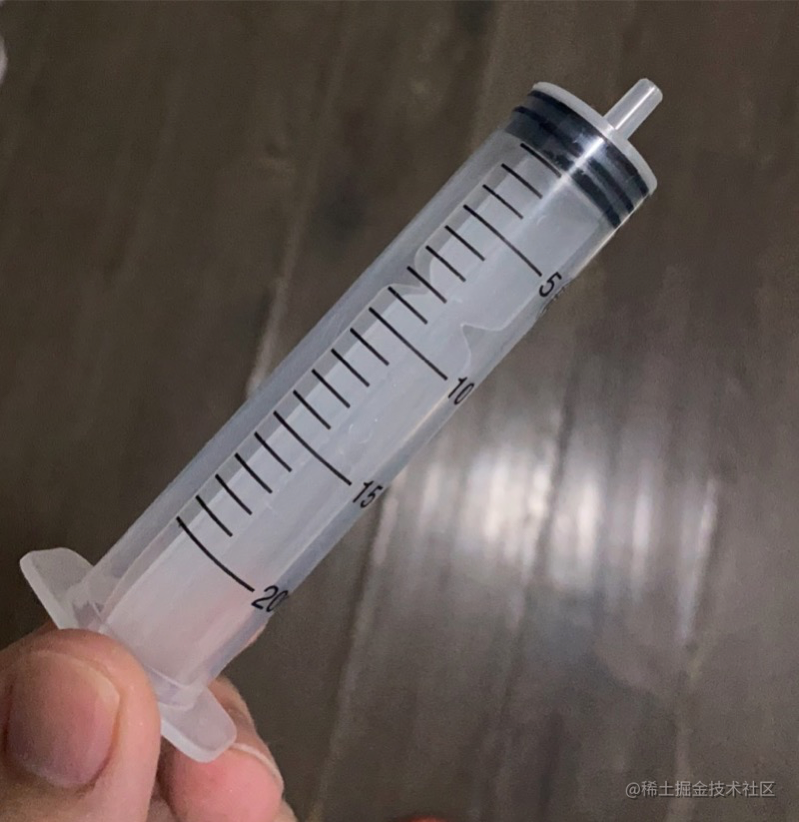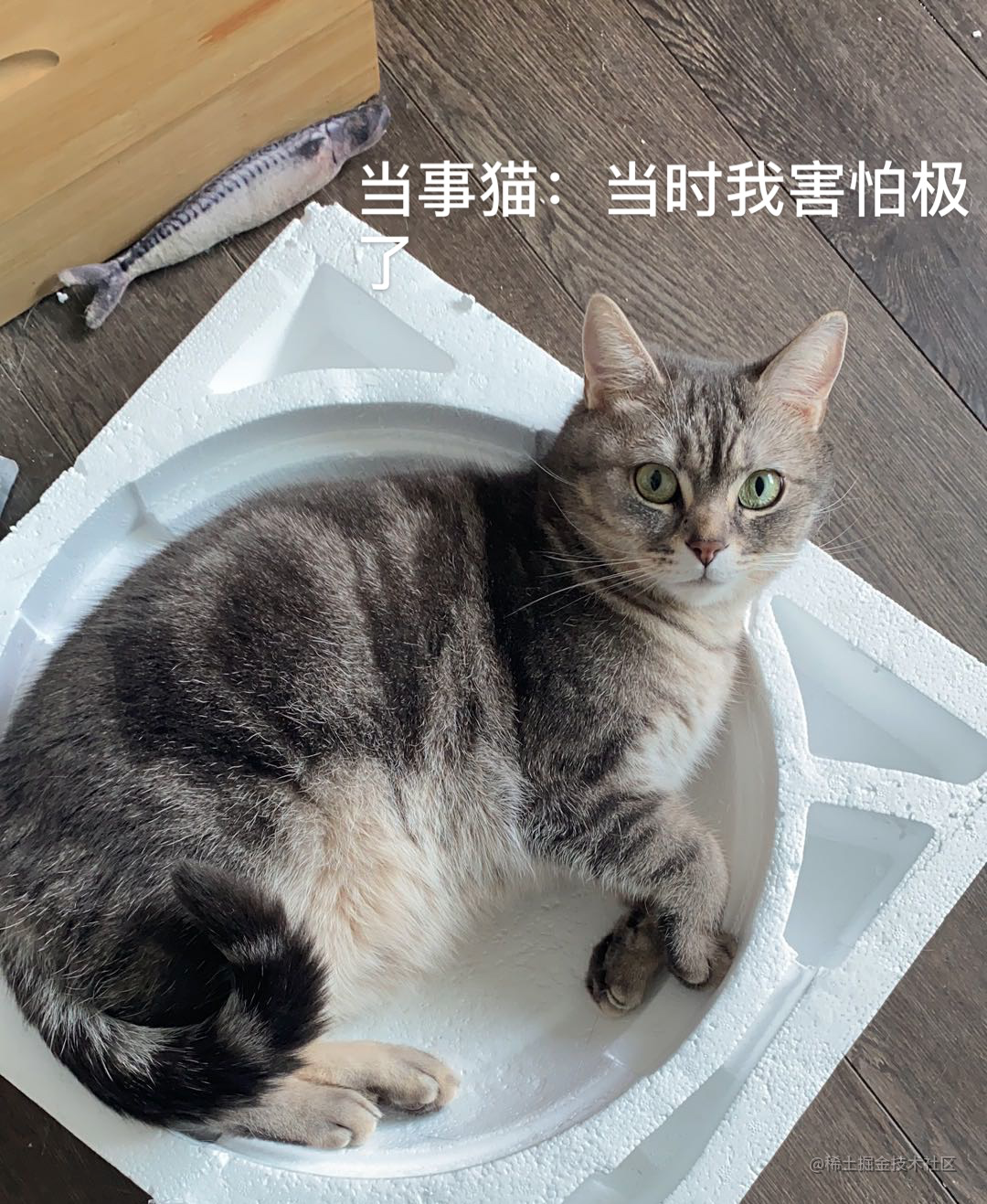👨：你也有脸说你知道你自己的身价吗？？？

#### 硬件设备准备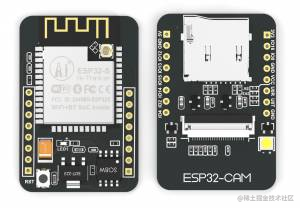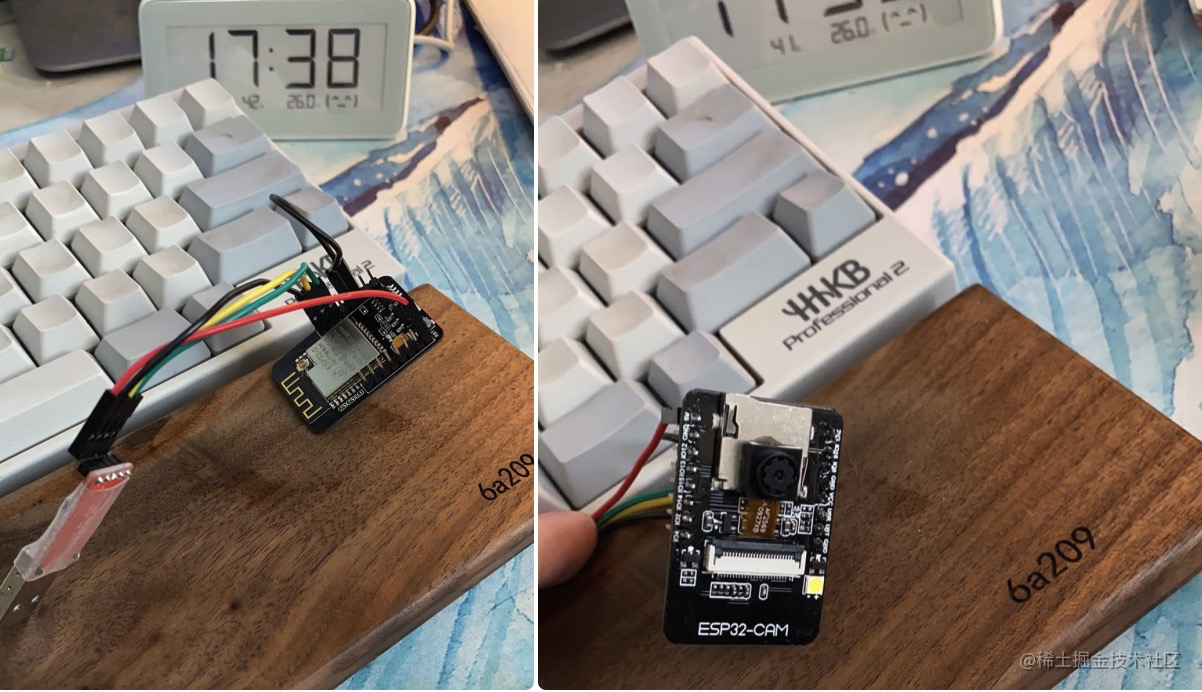#### 服务端设备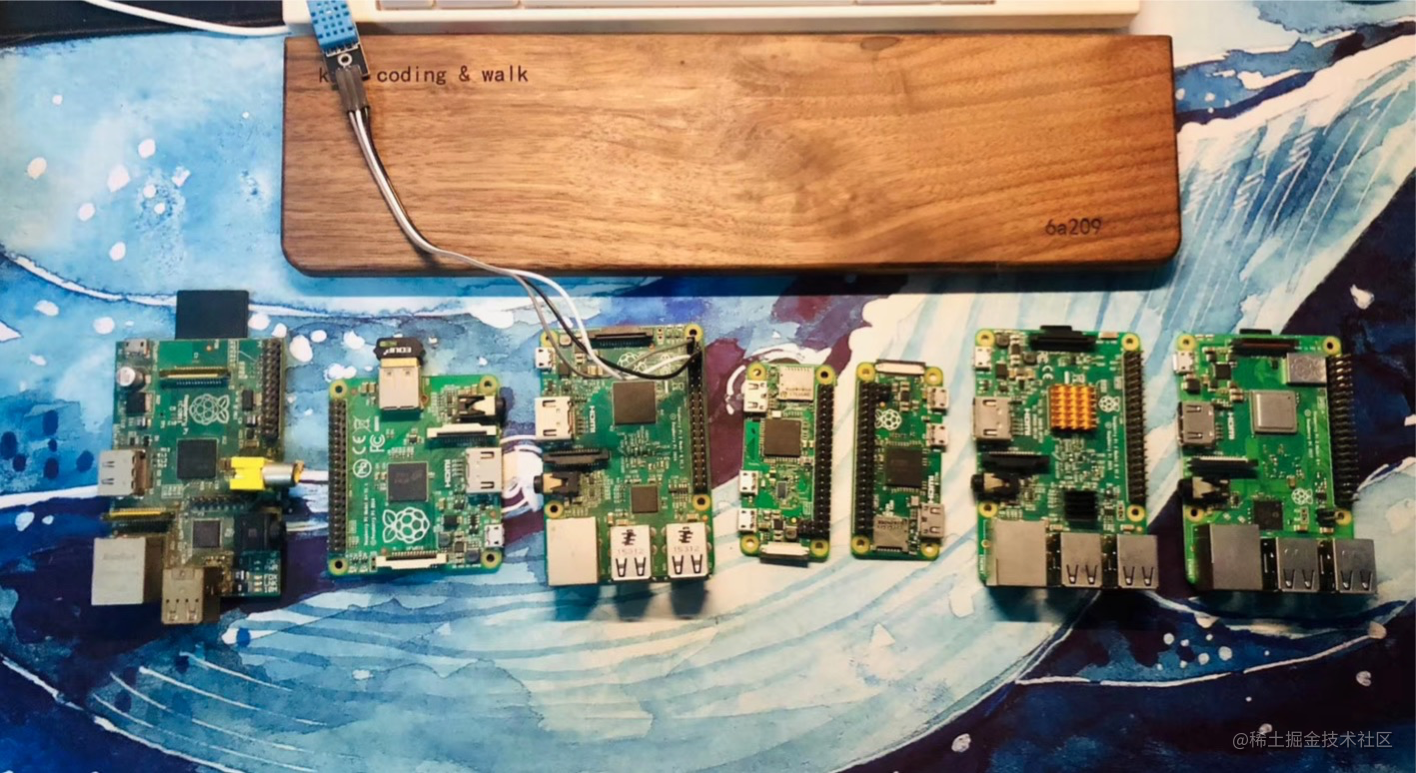#### 软件部分方案

AI部分采用Pytorch， 模型的话可以考虑用MobileNet 或者 SqueezeNet，

#### 设备端

``````    camera_config_t config;
config.ledc_channel = LEDC_CHANNEL_0;
config.ledc_timer = LEDC_TIMER_0;
config.pin_d0 = Y2_GPIO_NUM;
config.pin_d1 = Y3_GPIO_NUM;
config.pin_d2 = Y4_GPIO_NUM;
config.pin_d3 = Y5_GPIO_NUM;
config.pin_d4 = Y6_GPIO_NUM;
config.pin_d5 = Y7_GPIO_NUM;
config.pin_d6 = Y8_GPIO_NUM;
config.pin_d7 = Y9_GPIO_NUM;
config.pin_xclk = XCLK_GPIO_NUM;
config.pin_pclk = PCLK_GPIO_NUM;
config.pin_vsync = VSYNC_GPIO_NUM;
config.pin_href = HREF_GPIO_NUM;
config.pin_sscb_sda = SIOD_GPIO_NUM;
config.pin_sscb_scl = SIOC_GPIO_NUM;
config.pin_pwdn = PWDN_GPIO_NUM;
config.pin_reset = RESET_GPIO_NUM;
config.xclk_freq_hz = 20000000;
config.pixel_format = PIXFORMAT_JPEG;
config.frame_size = frameSize;
config.jpeg_quality = 10;
config.fb_count = 1;

bool ok = esp_camera_init(&config) == ESP_OK;

``````    camera_fb_t *frame_buffer = esp_camera_fb_get();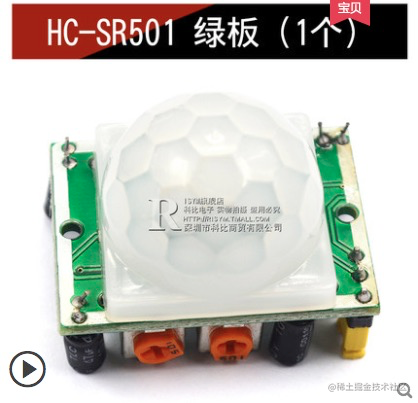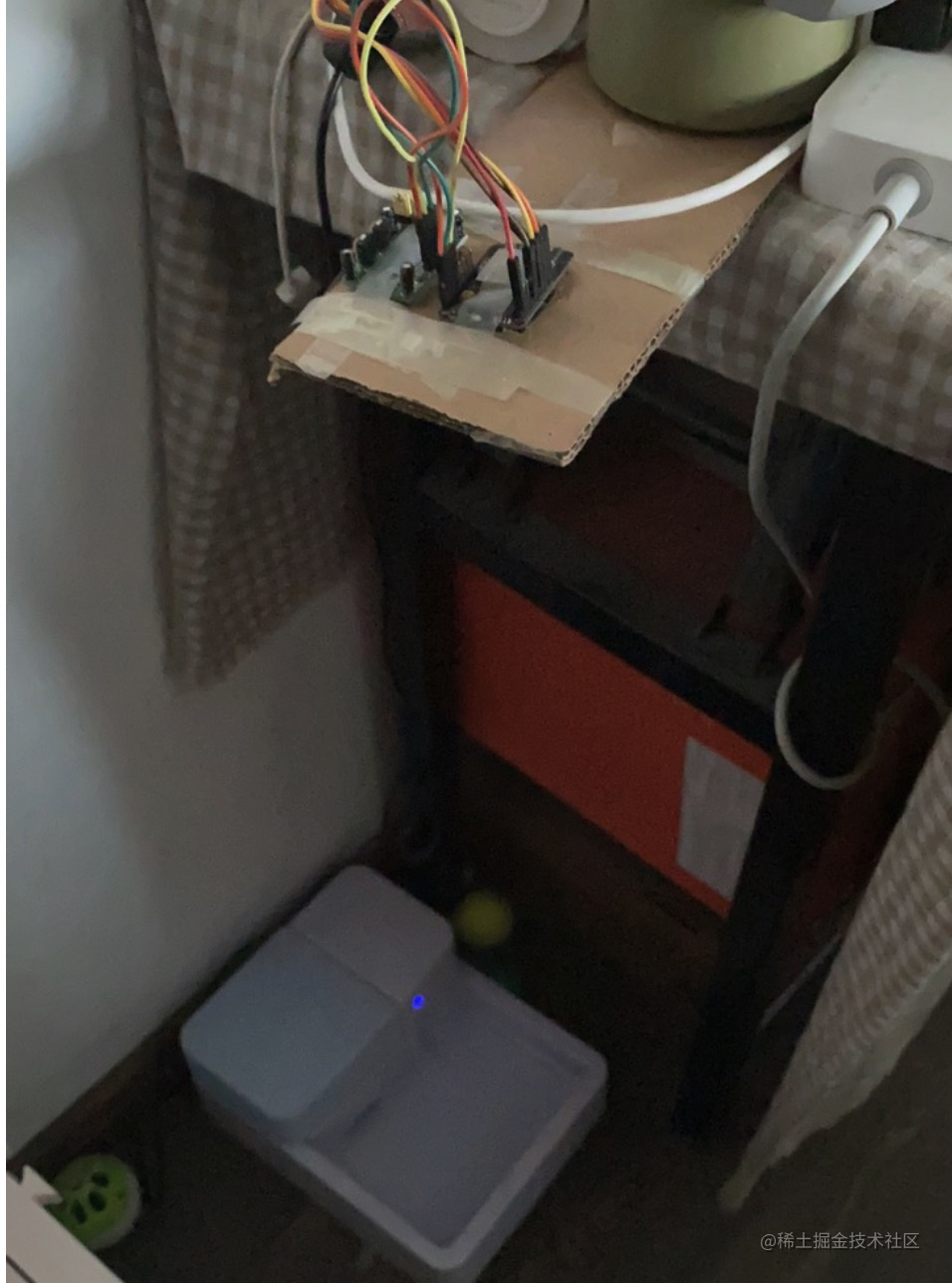ESP32 Cam 的 motion 检测

``````typedef enum {
PIXFORMAT_RGB565,    // 2BPP/RGB565
PIXFORMAT_YUV422,    // 2BPP/YUV422
PIXFORMAT_GRAYSCALE, // 1BPP/GRAYSCALE
PIXFORMAT_JPEG,      // JPEG/COMPRESSED
PIXFORMAT_RGB888,    // 3BPP/RGB888
PIXFORMAT_RAW,       // RAW
PIXFORMAT_RGB444,    // 3BP2P/RGB444
PIXFORMAT_RGB555,    // 3BP2P/RGB555
} pixformat_t;

1. 是计算量比较大
2. 是这样泛化能力比较差

``````        const uint16_t x = i % 240;
const uint16_t y = floor(i / 320);
const uint8_t block_x = floor(x / 10);
const uint8_t block_y = floor(y / 10);
const uint8_t pixel = frame_buffer->buf[i]
current_frame[block_y][block_x] += pixel;

### 服务端

``````  config.bodyParser = {
formLimit: '8mb',
enableTypes: ['json', 'form', 'text'],
extendTypes: {
text: ['*/*']
}
}

``````  async upload() {
const { ctx } = this;
const buffer = await getRawBody(ctx.req, { length: ctx.request.headers['content-length'] })
const time = sd.format(new Date(), 'YYYY-MM-DD_HH-mm-ss');
try {
fs.writeFileSync('./file-data/' + time + ".jpg", buffer);
} finally {
}
ctx.body = {
"code": 1001,
"msg": "ok"
}
}

### AI

##### 准备数据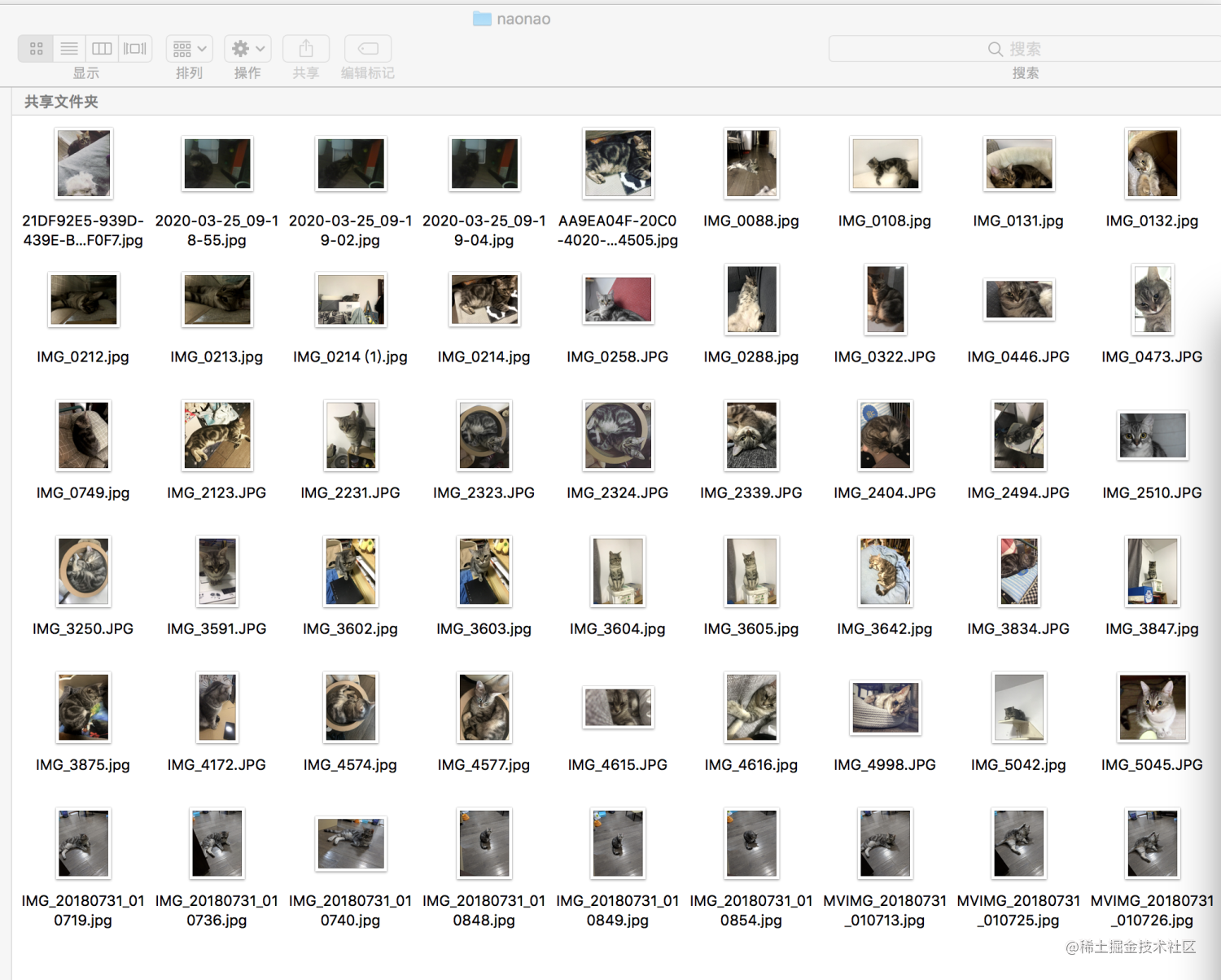##### 预处理数据

``````# coding:utf8
import os
from PIL import Image
from torch.utils import data
import numpy as np
from torchvision import transforms as T

class ChaochaoNaonao(data.Dataset):

def __init__(self, root, transforms=None, train=True, test=False):

self.test = test
imgs = []

for rootDir, dirs, files in os.walk(root):
for fileItem in files:
if "DS_Store" in fileItem:
continue
imgs.append(os.path.join(rootDir, fileItem))

imgs_num = len(imgs)
self.imgs = imgs
if transforms is None:
normalize = T.Normalize(mean=[0.485, 0.456, 0.406],
std=[0.229, 0.224, 0.225])

if self.test or not train:
self.transforms = T.Compose([
T.Resize(224),
T.CenterCrop(224),
T.ToTensor(),
normalize
])
else:
self.transforms = T.Compose([
T.Resize(256),
T.RandomResizedCrop(224),
T.RandomHorizontalFlip(),
T.ToTensor(),
normalize
])

def __getitem__(self, index):
"""
返回一张图片的数据
"""
img_path = self.imgs[index]
print(img_path)
if self.test:
label = int(img_path.split('/')[-1].split('.'))
else:
#  如果路径里有naonoa 定义为1 吵吵定义为 0
label = 1 if 'naonao' in img_path.split('/')[-2] else 0
data = Image.open(img_path)
data = self.transforms(data)
return data, label

def __len__(self):
return len(self.imgs)

##### 定义网络

``````from torchvision.models import  squeezenet1_1
from models.basic_module import  BasicModule
from torch import nn

class SqueezeNet(BasicModule):
# 修改为二分类
def __init__(self, num_classes=2):
super(SqueezeNet, self).__init__()
self.model_name = 'squeezenet'
self.model = squeezenet1_1(pretrained=True)
self.model.num_classes = num_classes
self.model.classifier = nn.Sequential(
nn.Dropout(p=0.5),
nn.Conv2d(512, num_classes, 1),
nn.ReLU(inplace=True),
nn.AvgPool2d(13, stride=1)
)

def forward(self,x):
return self.model(x)

def get_optimizer(self, lr, weight_decay):

##### 加载数据训练
``````  # train
for epoch in range(opt.max_epoch):

# train model
input = data.to(opt.device)
target = label.to(opt.device)

score = model(input)
loss = criterion(score,target)
loss.backward()
optimizer.step()

if (ii + 1)%opt.print_freq == 0:
vis.plot('loss', loss_meter.value())

# 进入debug模式
if os.path.exists(opt.debug_file):
import ipdb;
ipdb.set_trace()
print("loss : %s " %  loss_meter.value())

model.save()

epoch 50次后最终loss在0.2左右也不在拟合效果如何直接测试吧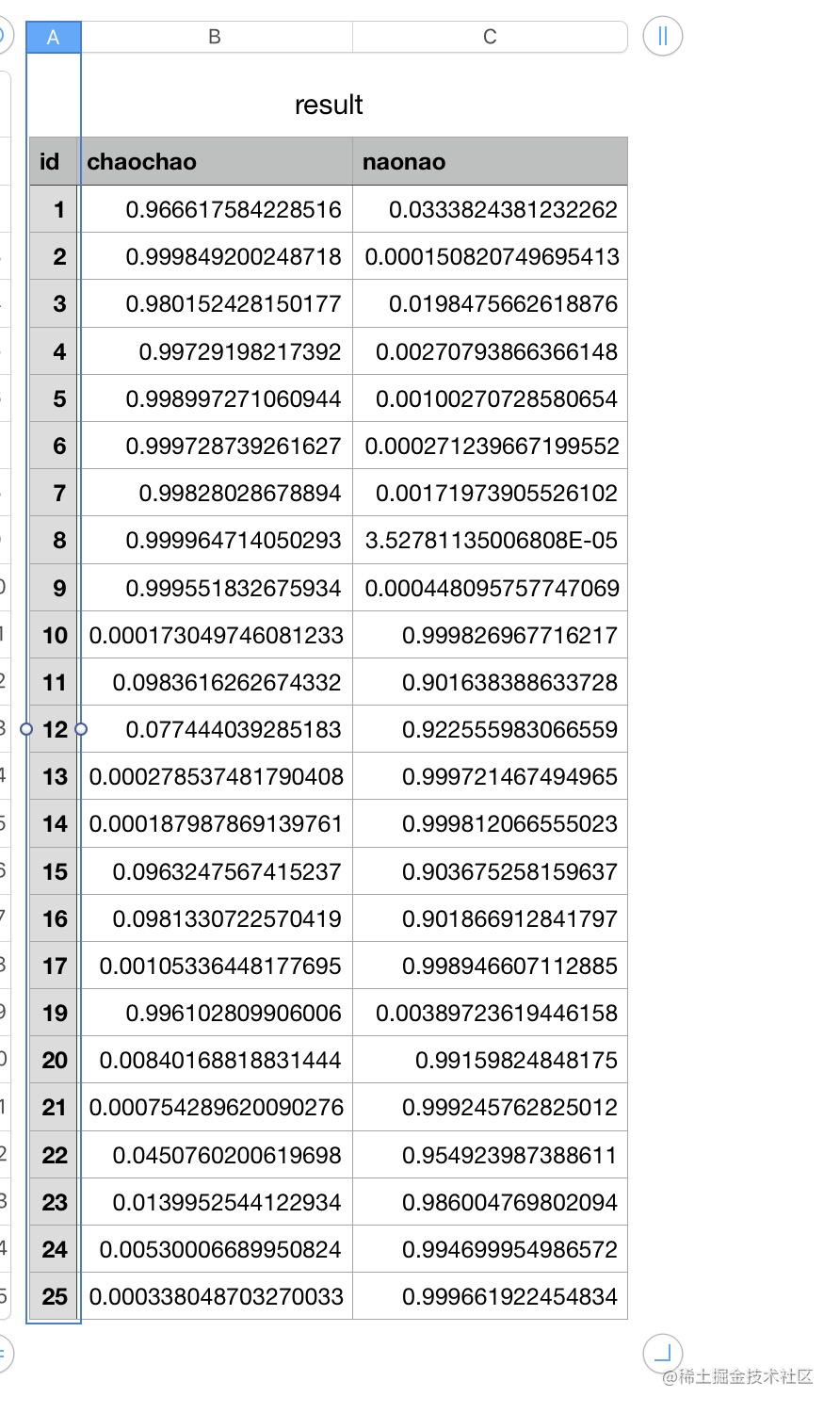### App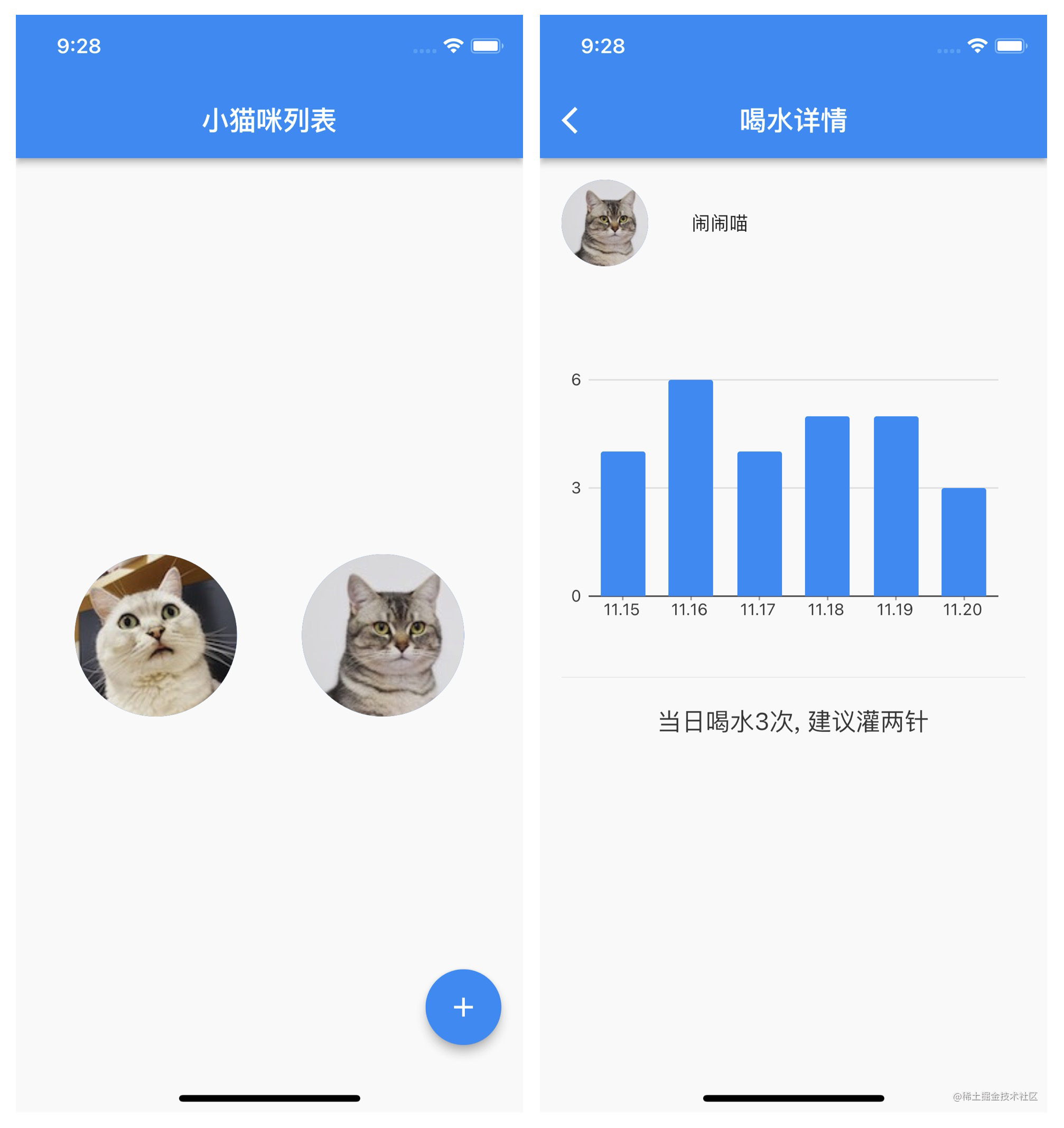### 尾声

👩：医生都说了这只猫子不喝水你做这个有什么用能让它多喝水吗？？

### 参考

github.com/fengdu78/Co…

github.com/chenyuntc/p…

github.com/espressif/e…

eggjs.org/zh-cn/

flutter.dev/